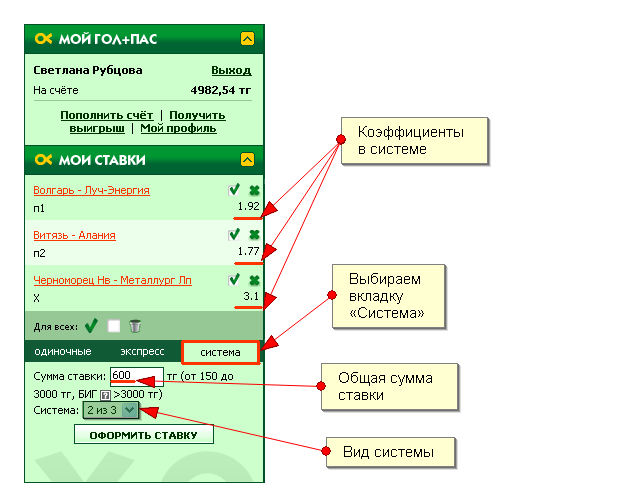0

# "Permutation" Bet

Various [single bets] (/help/stavka/) can be grouped, which enables you to increase the odds in case of a successful outcome. There are two types of betting combinations [Parlay] (/help/express/) and Permutation.

Permutation is essentially a combination of Parlay bets. Each Parlay included in the Perm bet consists of a certain number of coefficients.

How is a Perm bet combined on golpas.com website? You have to make a forecast on three or more outcomes (selecting 3 or more coefficients in the Line, maximum-16 coefficients), then select the "Perm" tab. Selecting more than three coefficients, you can also choose the Perm type, for example, "2 of 4" or "3 of 4". Permutation types will appear automatically under the field for entering the bet amount.

As an example, let’s review a simple Perm bet "2 of 3". It includes three events that are combined with each other by two in all possible ways (first with the second, first to the third, second with third). The amount wagered on each of these combinations is equal to 1/3 of the bet amount you have entered. In other types of Perm bets the bet amount is divided by the number of combinations in accordance with Perm Bet Type Table placed in [bookmaker's rules] (/rules/2/).

If we represent the system in the form of a formula, then in general it will look like this:C1, C2, C3 – event odds

Cs – the Perm odds

Maximum payout (W) will be:B – bet amount

Example:

To create a Perm bet select several events in [Line] (/account/line /), for example, three events:

1. «Volgar-Luch Enegria» W1, odds – 1.92;
2. "Chornomorets-Metallurg" X, odds – 3.1;
3. "Vityaz-Alania", W2, odds – 1.77.Next, choose the Perm type. Three double Parlays are created:

1. "Volgar" wins and "Alania" wins, odds. - 1.92×1.77;
2. Vctory of "Volgar" and a draw in "Chornomorets-Metallurg", odds. - 1.92×3.1;
3. Draw in "Chornomorets-Metallurg" and "Alania" wins, odds. - 3.1×1.77.

If total bet amount of KZT600, if all three of the events actually occur, the winning amount is:

1.92 & #215; 1.77 & #215; 200 + 1.92 & #215; 3.1 & #215; 200 + 3.1 & #215; 1.77 & #215; 200 = 14.83 & #215; 200 = 2967 KZT.

If one of the events is not triggered (for example, Volgar doesn't win), the automatically lost coefficient will become zero, which means that the calculation will be made as follows:

0×1.77×200 + 0×3.1×200 + 3.1×1.77×200 = 0 + 0 + 5.5×200 = 1100 KZT.

To win in such a Perm, it is enough to make two out of three (hence the name-"2 of 3") predictions prove to be correct. But at the same time it should be taken into account, that each of the Perm bet Parlays is only one third of the total bet amount, the winnings for such a Perm will be less than the possible total winnings. In addition, in some cases, when not all outcomes are guessed, the winning amount may be less than the bet amount. But unlike the multiple bet type, part of the bet amount will be reimbursed due to the guessed outcomes.

Please note that the bet amount is divided not by the number of events in the Perm, but by the number of Parlay options, which are created when the forming a Perm type bet. You can learn the Paraly options depending on how many events are there in the Perm in the "Perm Bet Type Table" in the Rules section ["Betting types and combinations"] (/rules/4/). You can also use [Calculator] (http://golpas.com/calc/).

Go back to ["Help]section (/help/).

• No odd in the current betting line (l)
• No odd in the current betting line (l)
• Np odd in the current betting line (u)
• Odds admission time is out
• The event does not allow to place a bet
• The odd is temporarily out of the betting line
• Deleted by user
• Internal error
• The amount of the bet can not be less than 0
• Exceeded maximum bid amount
• The cart can not contain more than 0 события
• No bet amount specified
• Invalid value entered «x:x».
• You must enter numeric values in the fields
• Это БИГ-ставка, будет ожидать подтверждения администратора
• В соответствии с Правилами бонусной программы после использования бонусного счёта будут введены ограничения на вывод средств. Подтвердите ставку с бонусного счёта
• Слишком низкий коэффициент. Для ставки с бонусного счета коэффициент должен быть >=
• Один или несколько коэффициентов в составе квитанции ниже указанных в правилах ()
• Одиночные ставки нельзя оплатить с бонусного счета
• Слишком мало событий для оплаты с бонусного счета
• To place a bet you have to logg in
• Your balance is low
• Betting is temporarily suspended
• No odds selected
• Bet parameters must be specified
• Changes occurred in the cart. If you agree - click "Verify"
• Accumulator must contain at least 2 events
• Accumulator can not contain dependent odds
• Invalid system type for a given set of odds.
• The system can not contain dependent odds
• The system can not consist of less than three events
• Максимальное количество позиций типа ставки "показатели игроков" в экспрессе -
• Insufficient permissions for this operation
• Maximum bid amount
• The maximum amount of payout at this bet . Reduce your bet to .
• Bet declined